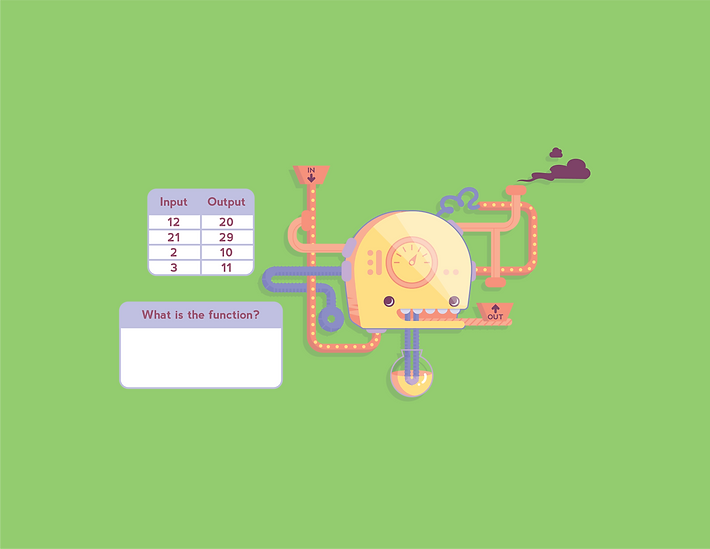## FUNCTIONS

Growing IQ students are first introduced to the concept of function through the exploration of arithmetic and geometric sequences.  As knowledge and familiarity increase, the students are introduced to function equations which contain two unknown variables (represented by x and y and can be graphed in a coordinate system).

Functions are represented in a multitude of areas of mathematics including algebraic operations, sequences, probability models, graphs, and calculus.## 3 Categories of Functions## INPUT/OUTPUT

Functions can be explored through function machines, where an input quantity goes in and a different quantity comes out.  Analyzing the inputs and outputs introduces parameters and brings to light the mystery function rule that the machine is using to transform each number.## Graphing functions creates a meaningful representation of the set of ordered pairs (inputs and outputs) allowing visualization of the the algebraic relationships present in a function.  Graphing functions allows the evaluation of expressions, comparison of functions, and discussion of the various properties within the context of a problem.## EXPONENTIAL

Exponential functions are non-linear and describe examples of continuous growth or decay.  Exponential functions lead to calculus and applications in chemistry, biology, and physics where various relationships are expressed in terms of accelerating and decelerating factors.